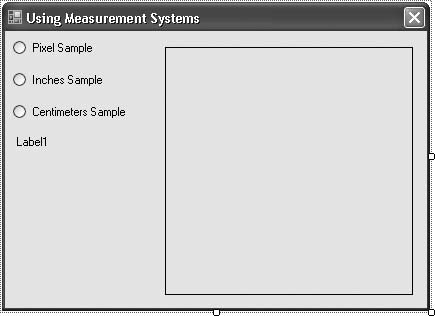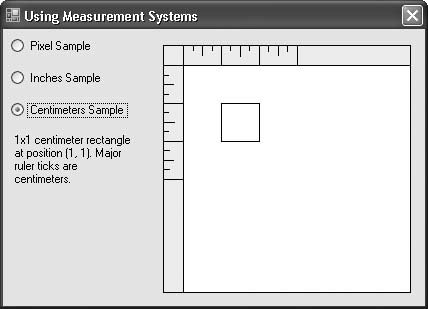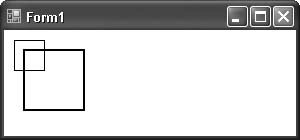# Recipe 9.4. Working with Coordinate Systems (Pixels, Inches, Centimeters)

#### Problem

You've been drawing on a graphics canvas (such as the surface of a form or control), and working with pixels. But your program lets the user work in inches or centimeters, and you don't want to do all the conversions yourself.

#### Solution

Sample code folder: Chapter 09\MeasurementSystems

The Graphics object that you receive in a Paint event handler (or that you create else-where) provides a few different ways to scale to different measurement systems. The easiest way is to set its PageUnit property to one of the predefined GraphicsUnit enumeration values. The sample code in this recipe uses GraphicsUnit.Display (the default), .Inch, and .Millimeter.

#### Discussion

Create a new Windows Forms application, and add the following controls to Form1:

• A RadioButton control named ShowPixels. Set its Text property to Pixel Sample.

• A RadioButton control named ShowInches. Set its Text property to Inches Sample.

• A RadioButton control named ShowCentimeters. Set its Text property to Centimeters Sample.

• A Label control named Comment. Set its AutoSize property to False, and resize it so that it can hold a dozen or so words.

• A PictureBox control named SampleDisplay. Set its BorderStyle property to FixedSingle. Size it at about 250 x 250 pixels.

Your form should look something like Figure 9-5.

Now add the following source code to the form's class template:

` Private Sub ChangeSystem(ByVal sender As System.Object, _       ByVal e As System.EventArgs) _       Handles ShowPixels.CheckedChanged, _       ShowInches.CheckedChanged, _       ShowCentimeters.CheckedChanged    ' ------ Update the example text.    If (ShowPixels.Checked = True) Then       Comment.Text = "50x50 rectangle at position " & _          "(50, 50). Major ruler ticks are at 100 pixels."    ElseIf (ShowInches.Checked = True) Then       Comment.Text = "1x1 inch rectangle at position " & _          "(1, 1). Major ruler ticks are inches." `

##### Figure 9-5. The controls in the measurement systems sample`    Else       Comment.Text = "1x1 centimeter rectangle at " & _          "position (1, 1). Major ruler ticks are centimeters."    End If    ' ----- Now update the display.    SampleDisplay.Invalidate() End Sub Private Sub Form1_Load(ByVal sender As Object, _       ByVal e As System.EventArgs) Handles Me.Load    ' ----- Show the pixel example by default.    ShowPixels.Checked = True End Sub Private Sub SampleDisplay_Paint(ByVal sender As Object, _       ByVal e As System.Windows.Forms.PaintEventArgs) _       Handles SampleDisplay.Paint    ' ----- Draw the surface based on the user's selection.    Dim rectangleArea As Rectangle    Dim thinPen As Pen    Dim rulerWidth As Single    Dim tickStep As Single    Dim tickSize As Single    Dim counter As Integer    Dim bigTick As Single    Const ticks As String = "1424142414241"    ' ----- Clear any previous content.    e.Graphics.Clear(Color.White)    ' ----- Adjust to the right system.    If (ShowPixels.Checked = True) Then       ' ----- Draw a 50-by-50-pixel rectangle at (50,50).       rectangleArea = New Rectangle(50, 50, 50, 50)       rulerWidth = e.Graphics.DpiX / 5.0F       bigTick = 100.0F    ElseIf (ShowInches.Checked = True) Then       ' ----- Scale for inches.       e.Graphics.PageUnit = GraphicsUnit.Inch       ' ----- Draw a 1" x 1" rectangle at (1,1).       rectangleArea = New Rectangle(1, 1, 1, 1)       rulerWidth = 0.2F       bigTick = 1.0F    Else       ' ----- Scale for centimeters (actually, millimeters).       e.Graphics.PageUnit = GraphicsUnit.Millimeter       ' ----- Draw a 1cm x 1cm rectangle at (1,1).       '       Note: 0.2 inches is 1/5 of 25.4 millimeters.       rectangleArea = New Rectangle(10, 10, 10, 10)       rulerWidth = 25.4F / 5.0F       bigTick = 10.0F    End If    ' ----- Create a single-pixel pen.    thinPen = New Pen(Color.Black, 1 / e.Graphics.DpiX)    ' ----- Draw a ruler area. The rulerWidth is 0.2 inches    '       wide, no matter what the scale. Make a 3-inch    '       ruler.    e.Graphics.FillRectangle(Brushes.BlanchedAlmond, 0, 0, _       rulerWidth, rulerWidth * 15)    e.Graphics.FillRectangle(Brushes.BlanchedAlmond, 0, 0, _       rulerWidth * 15, rulerWidth)    e.Graphics.DrawLine(thinPen, rulerWidth, rulerWidth, _       rulerWidth, rulerWidth * 15)    e.Graphics.DrawLine(thinPen, rulerWidth, rulerWidth, _       rulerWidth * 15, rulerWidth)    ' ----- Draw the ruler tick marks. Include whole steps,    '       half steps, and quarter steps.    For counter = 1 To ticks.Length       ' ----- Get the tick measurements. The "ticks" constant       '       includes a set of "1", "2", and "4" values. "1"       '       gives a full-size tick mark (for whole units),       '       "2" gives a half-size tick mark, and "4" gives       '       a 1/4-size tick mark.       tickSize = CSng(Mid(ticks, counter, 1))       tickStep = rulerWidth + ((bigTick / 4.0F) * (counter - 1))       ' ----- Draw the horizontal ruler ticks.       e.Graphics.DrawLine(thinPen, tickStep, 0, _          tickStep, rulerWidth / tickSize)       ' ----- Draw the vertical ruler ticks.       e.Graphics.DrawLine(thinPen, 0, tickStep, _          rulerWidth / tickSize, tickStep)    Next counter    ' ----- Adjust the (0,0) point to the corner of the ruler.    e.Graphics.TranslateTransform(rulerWidth, rulerWidth)    ' ----- Draw the rectangle.    e.Graphics.DrawRectangle(thinPen, rectangleArea)    ' ----- Put things back to normal.    e.Graphics.PageUnit = GraphicsUnit.Display    thinPen.Dispose() End Sub `

Run the program, and click on each of the three radio buttons to see the results. Figure 9-6 shows the application using centimeters.

##### Figure 9-6. Drawing using centimeters (millimeters) as the unit systemThe focus of the application is on drawing the black rectangle:

` e.Graphics.DrawRectangle(thinPen, rectangleArea) `

The rest of the code is there to make it easy to see the difference between the drawing systems.

The Graphics object defaults to the coordinate system of the display. On a monitor, each unit is a single pixel. When you draw a 10 x 10 rectangle, you are drawing a rectangle 10 pixels high and 10 pixels wide. To draw a 10 x 10-inch rectangle, you need to change the scaling system so that "1" represents an inch instead of a pixel.

The PageUnit property does just that. It supports a few common measurement systems, including Inches, Millimeters, and even Points.

You can also create your own custom scaling factor in each direction (X and Y) by using the Graphics object's ScaleTransform() method. This lets you set a scaling factor for both the horizontal (X) and vertical (Y) directions. To see scaling in action, create a new Windows Forms application, and add the following source code to the form's code template:

` Private Sub Form1_Paint(ByVal sender As Object, _       ByVal e As System.Windows.Forms.PaintEventArgs) _       Handles Me.Paint    e.Graphics.Clear(Color.White)    e.Graphics.DrawRectangle(Pens.Black, 10, 10, 30, 30)    e.Graphics.ScaleTransform(2, 2)    e.Graphics.DrawRectangle(Pens.Black, 10, 10, 30, 30) End Sub `

This code draws two 30 x 30 rectangles, one normal (i.e., 30 x 30 pixels), and one scaled by a factor of two in each direction (resulting in a 60 x 60 square). Figure 9-7 shows the output of this code.

##### Figure 9-7. A normal and a scaled squareEverything about the second (larger) square is scaled by two: its size, its starting position (at (20,20) instead of (10,10)), and even the thickness of its pen (it's twice as thick).Visual Basic 2005 Cookbook: Solutions for VB 2005 Programmers (Cookbooks (OReilly))
ISBN: 0596101775
EAN: 2147483647
Year: 2006
Pages: 400

Similar book on Amazon